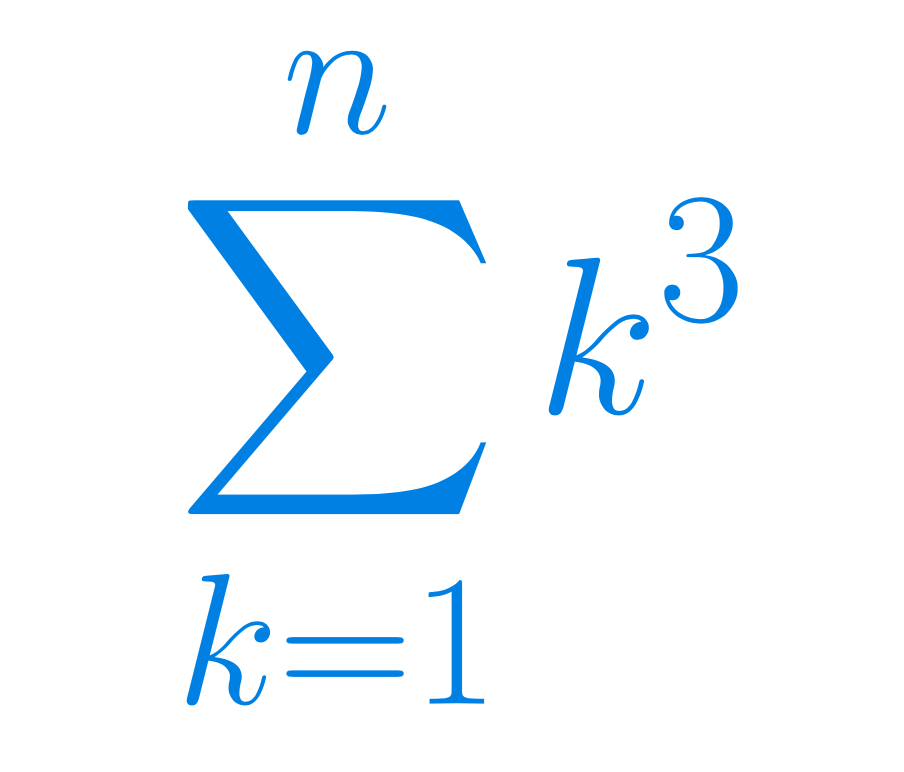Select Page

# Fun tricks for everybody

#### A two hour long free course on fun tricks in mathematics#### Prerequisites

High-school maths, mainly arithmetic.

#### Curriculum

This course concentrates on a few interesting and fun examples of derivations of formulas and their geometrical interpretation.

# Math: Fun tricks for everybody

h

## Get the outline

A detailed list of the lectures can be found in a PDF outline by clicking this panel.## Get Fun tricks for everybody

This course is completely free on Udemy. Click this panel to get to the download page.

## Course Objectives & Outcomes

Z
Creative problem solving, with fun tricks and geometrical illustrations of the results.
Z
How to derive the formulas for partial sums for two very important examples of arithmetic progressions: 1+2+…+n and 1+3+5+…+(2n-1).
Z
How to derive some less known formulas, using cool computational tricks.
Z
You will learn (from an article) how to convert infinite periodic decimal expansions into fractions, by smart substitutions and solving linear equations.
Z
You will learn (from an article) about an interesting family of nested square roots and how to prove that some members of this family are… integers!
Z
How to derive the formula for the sum of squares of all natural numbers from 1 to n.
Z
How to derive the general formula for partial sums of geometric progressions; an important example of the sum of all the natural powers (from 1 to n) of 1/2.
Z
How to prove divisibility in some simple cases, using very basic (but fun) reasoning.
Z
You will learn (from an article) some basics about continued fractions (CF), and how to recognise irrational surds from their infinite periodic CF-expansions.